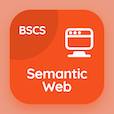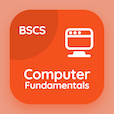Computer Science Courses Online

Digital Logic Design Exam Prep

Digital Logic Design Practice Test 127

The e-Book Adder and Subtractors Quiz Questions, adder and subtractors MCQ with answers PDF chapter 8-127 to learn online courses, digital logic design tests. Practice DLD Lab Equipment and Experiments trivia questions, adder and subtractors Multiple Choice Questions (MCQ Quiz) for online college degrees. The e-Book "Adder and Subtractors Quiz" App Download: adder and subtractors, special characteristics of circuits, introduction to digital circuits test prep for computer software engineer online degree.

The Quiz "Subtraction of two numbers can be done using" PDF, Adder and Subtractors App Download (Free) with adder, adder-subtractor, divider, and multiplier choices for computer software engineer. Solve dld lab equipment and experiments questions and answers, Amazon eBook to download free sample to learn online certificate courses.

## Logic Design: Adder & Subtractors Quiz

MCQ: Subtraction of two numbers can be done using

C) divider
D) multiplier

MCQ: The standard value of Vil in noise margin is

A) 0.2V
B) 0.3V
C) 0.5V
D) 0.8V

MCQ: Metal Oxide Semiconductor (MOS) is being used in

A) LSI
B) VLSI
C) MSI
D) both a and b

MCQ: Symbolic notation A← B represents

A) Clear Register
B) Set Flip-flop
C) Increment register A
D) Transfer contents of register B into A

MCQ: Noise margin is calculated by

A) Voh+Vih
B) Vh-Vi
C) Voh-Vih
D) VohxVih

### Mock Tests: Digital Logic Design Course Prep

Download Digital Logic Design Quiz App, Semantic Web MCQs App and Computer Fundamentals MCQ App for Android & iOS devices. These Apps include complete analytics of real time attempts with interactive assessments. Download Play Store & App Store Apps & Enjoy 100% functionality with subscriptions!